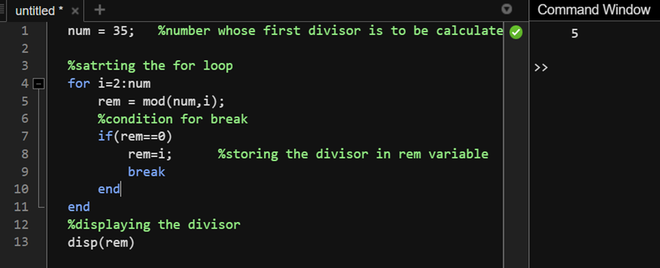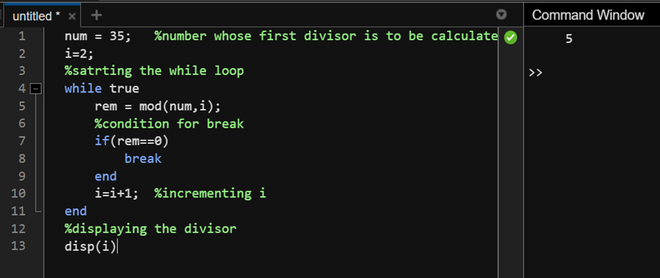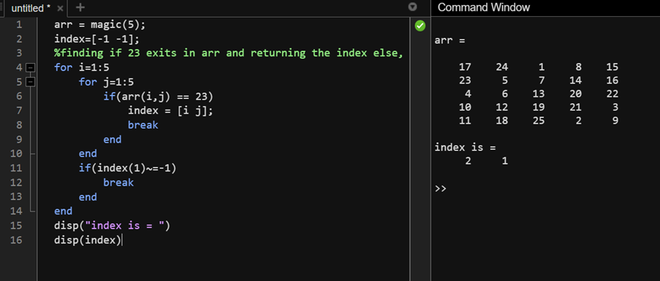Open in App
Not now

# MATLAB – Break Statement

• Last Updated : 21 Nov, 2022

Break statement in MATLAB is used for breaking out of an iterative loop, for or while loop. The break in MATLAB is similar to the break statements in other programming languages such as C, C++, Python, etc. We will see how to break out of a single for or while loop and the nested implementation of the same.

### Syntax:

• For loop

for <iteration condition>

statement 1

————–

break conditional

break

end

• While loop

while <condition>

statement 1

————–

break conditional

break

end

We will use a loop that returns the first complete divisor of a number in the specified range.

Example 1:

S

Output:We will use the same case with the while loop to see the usage of a break in the while loop.

Example 2:

## Matlab

 `% MATLAB code for break in the while loop.``% Number whose first divisor is to be calculated``num = 35; ``i=2;` `%starting the while loop``while` `true``    ``rem = mod(num,i);``    ` `    ``% Condition for break``    ``if``(rem==0)``        ``break``    ``end``    ``%incrementing i``    ``i=i+1; ``end` `% Displaying the divisor``disp(i)`

Output:Using the break statement with nested loops.

We will find the index of number 23 in magic square of 5×5 using nested loops.

Example 3:

## Matlab

 `% MATLAB code for update``arr = magic(5);``index=[-1 -1];` `% Finding if 23 exits in arr and returning the index else, return -1``for` `i=1:5``    ``for` `j=1:5``    ``% Checks if arr(i,j) is 23``        ``if``(arr(i,j) == 23)   ``            ``index = [i j];   ``            ``break`       `            ``% Breaks the inner loop if 23 is found``        ``end``    ``end``    ``% Checks if value of row is changed``    ``if``(index(1)~=-1)   ``        ``% Breaks the outer loop if row is not -1``        ``break`           `    ``end``end``% Displays the index of 23 in magic(5)``disp(``"index is = "``)``disp(index)`

Output:As can be seen, 23 is located in the 1st column of the 2nd row thus, the index is 2,1.

My Personal Notes arrow_drop_up